# GMAT Math : Calculating whether lines are perpendicular

## Example Questions

2 Next →

### Example Question #11 : Calculating Whether Lines Are Perpendicular

Which of the following lines is perpendicular to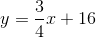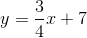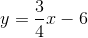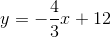Not enough information provided.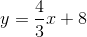Explanation:

Given a linedefined by the equationwith a slope of, any line perpendicular towould have a slope that is the negative reciprocal of,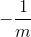. Given our equation, we know that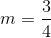and that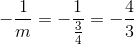The only answer choice with this slope is### Example Question #12 : Calculating Whether Lines Are Perpendicular

Which of the following lines is perpendicular to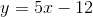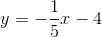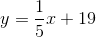Two of the answers are correct.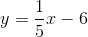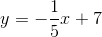Two of the answers are correct.

Explanation:

Given a linedefined by the equationwith a slope of, any line perpendicular towould have a slope that is the negative reciprocal of. Given our equation, we know that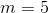and that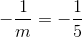There are two answer choices with this slope,and.

### Example Question #13 : Calculating Whether Lines Are Perpendicular

A given lineis defined by the equation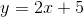. Which of the following lines would be perpendicular to line?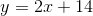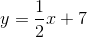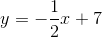Not enough information provided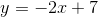Explanation:

For any linewith an equationand slope, a line that is perpendicular tomust have a slope of, or the negative reciprocal of. Given, we know that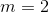and therefore know that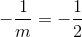Only one equation above has a slope of### Example Question #14 : Calculating Whether Lines Are Perpendicular

What is the slope of a line that is perpendicular to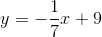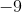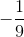Explanation:

For any linewith an equationand slope, a line that is perpendicular tomust have a slope of, or the negative reciprocal of. Given the equation, we know that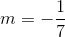and therefore know that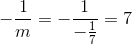.

### Example Question #15 : Calculating Whether Lines Are Perpendicular

Which of the following lines is perpendicular to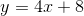?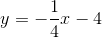None of the lines is perpendicular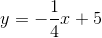Two lines are perpendicular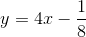Two lines are perpendicular

Explanation:

For any linewith an equationand slope, a line that is perpendicular tomust have a slope of, or the negative reciprocal of. Given the equation, we know that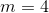and therefore know that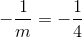Given a slope of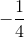, we know that there are two solutions provided:and### Example Question #16 : Calculating Whether Lines Are Perpendicular

What is the slope of a line perpendicular to that of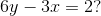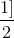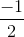Explanation:

First, we need to rearrange the equation into slope-intercept form..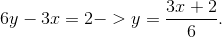Therefore, the slope of this line equals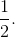Perpendicular lines have slope that are the opposite reciprocal, or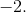2 Next →

### All GMAT Math Resources